# Understanding exponential growth: The corona pandemic

With the news going crazy these days, I felt like there is one particularly thing that is often misunderstood. The corona virus spreads exponentially (without intervention or measures). The problem is that we – as human beings – are very bad at imagining what an exponential trend looks like. By now, many differnet graphics and figures appear everywhere that aim to visualize the amount of infections or mortality rates per country. One of the most prominent examples is the following one:

Although this figure is not wrong, I would like to highlight one particular aspect. At first glance, the figure seems to suggest that the growth curves are “linear”. However, the devil is in the details. If you look closely, you realize that the y-axis is log-transformed. But was does that actually mean and why is it so important to understand such a procedure? In this post, I try to explain, show, and visualize exponential growth.

Disclaimer:
I would like to highlight that I am no epidemiologist or expert on virus outbreaks. My goal with this post is to explain exponential growth. My goal is not to provide a better understanding of the corona pandemic in general. Virologist, epidemiologist, and health care institutions are the experts that should be consulted in this regard.

# How does exponential growth actually look like?

In what follows, I define a small function that allows us to simulate an exponentially growing vector. This means that each value is the previous value multiplied by a certain factor:

x_i = x_{i-1}*factor
``````library(tidyverse)
library(papaja)

# Exponential function
exp_growth <- function(start = 1,
steps = 10,
factor = 2) {
x <- vector()
x <- start
for(i in 2:steps){
x[i] = x[i-1]*factor
}
return(x)
}

# Where are we after only 10 steps?
exp_growth()``````
``##     1   2   4   8  16  32  64 128 256 512``

If we run the function with the default values (starting value = 1, steps = 10, factor = 2), we reach the number 512 after only 10 steps. Impressive growth, right?

``````# Where are we after 20 steps?
exp_growth(steps = 20)``````
``````##        1      2      4      8     16     32     64    128    256    512
##    1024   2048   4096   8192  16384  32768  65536 131072 262144 524288``````

After only 20 steps, however, we already reach a number that is larger than 0.5 million.

To get a true understanding of exponential growth, let us visualize 30 steps (while still using a starting value of 1).

``````(plot1 <- data.frame(n = 1:30,
value = exp_growth(steps = 30)) %>%
ggplot(aes(x = n, y = value)) +
geom_point() +
geom_line() +
theme_bw())``````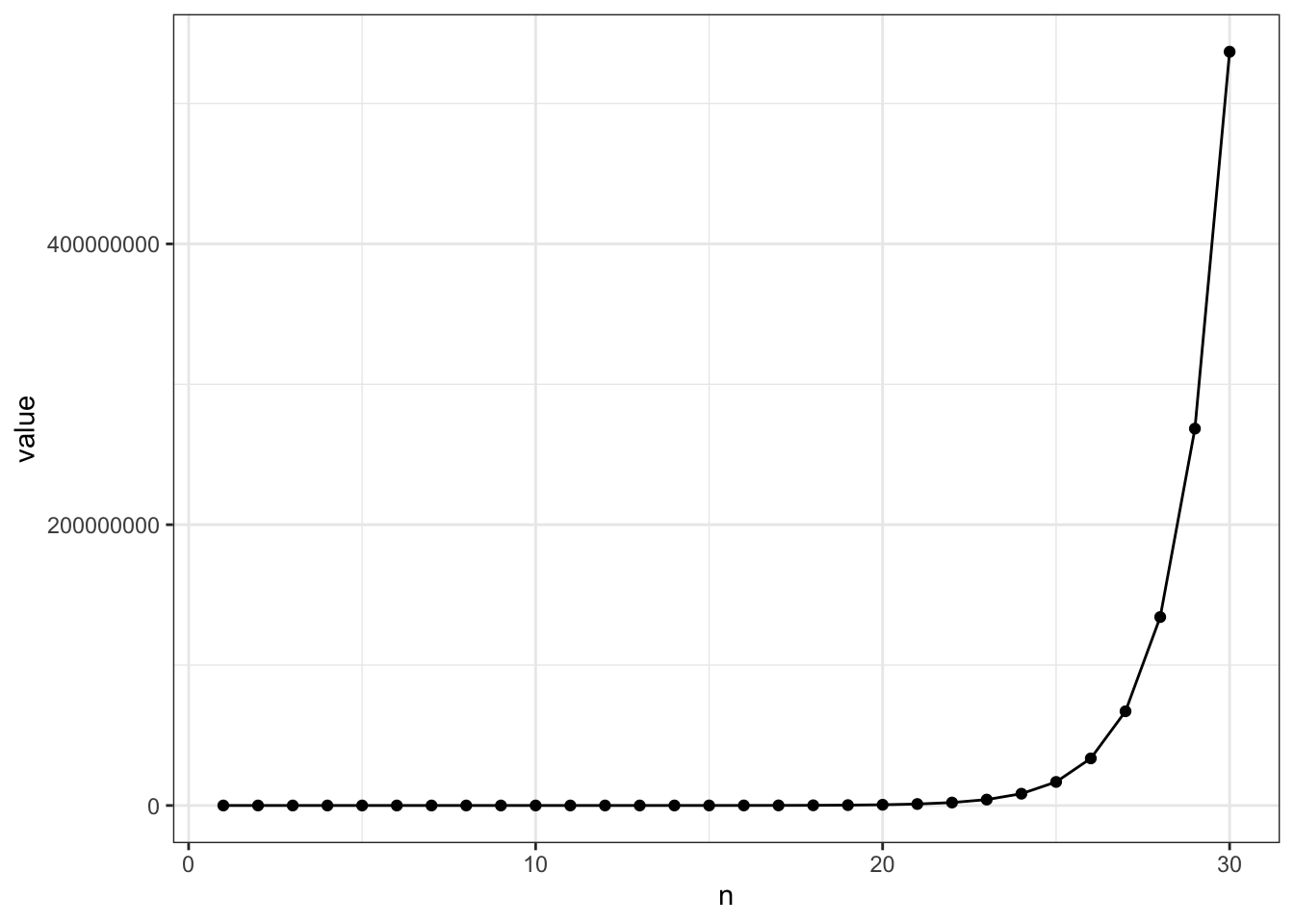After 30 steps only, we have reached the crazy large number of 536,870,912 (more than 500 million!). At the same time, it seems that the growth only really takes off after 25 steps. But remember our previous calculation. We were already at 0.4 million after 20 steps. So let’s zoom in on the first 10 steps again:

``````plot1 +
ylim(0,750) +
scale_x_continuous(breaks = c(1:10), limits = c(1,10))``````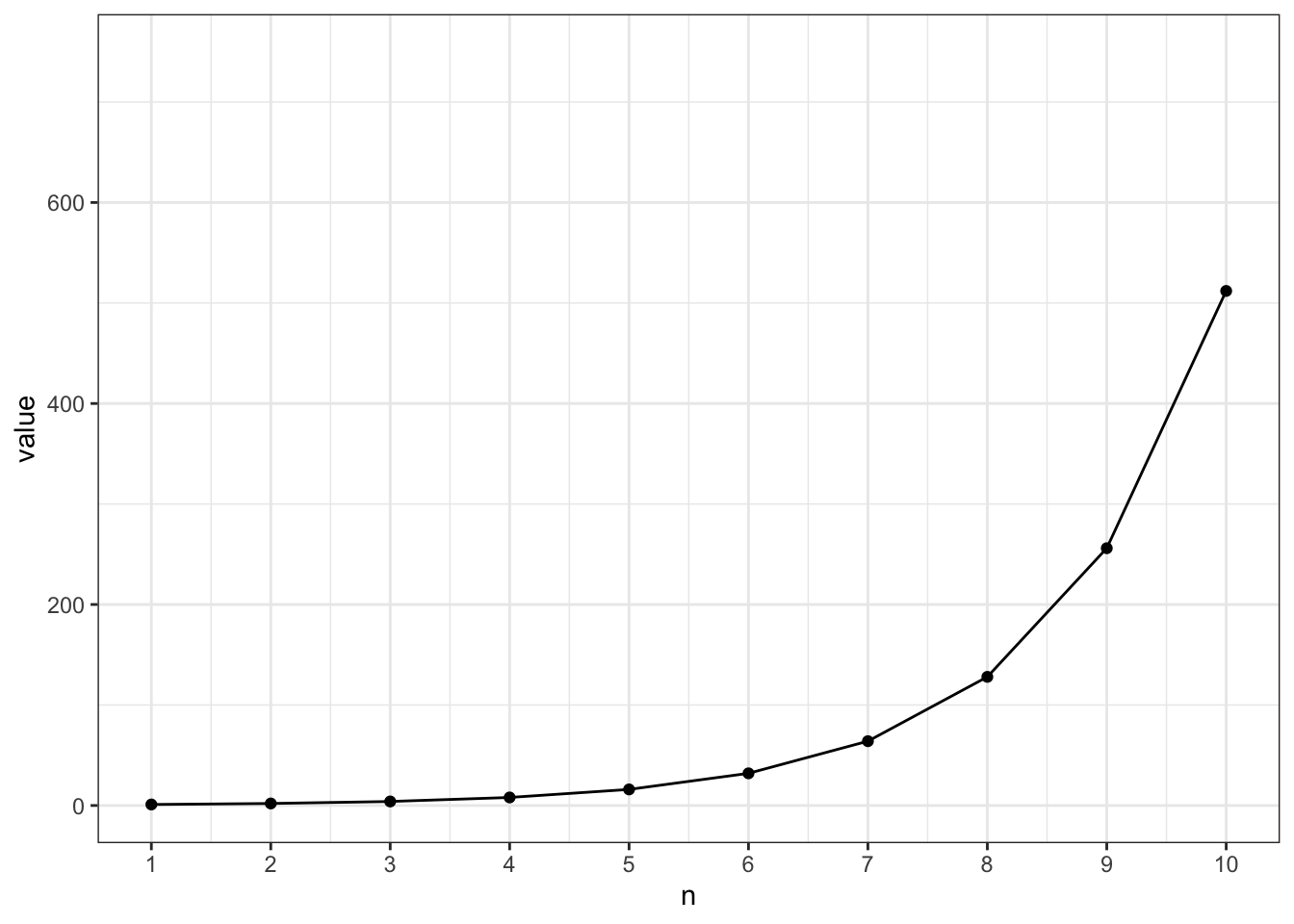After only 10 steps, we reached a number higher than 500. This steep growth was buried in the previous figure because the growth afterwards was just sooo much steeper.

No what happens if we logarithmize the y-axis (as it was done in the figure referenced above)?

``````plot1 +
scale_y_log10()``````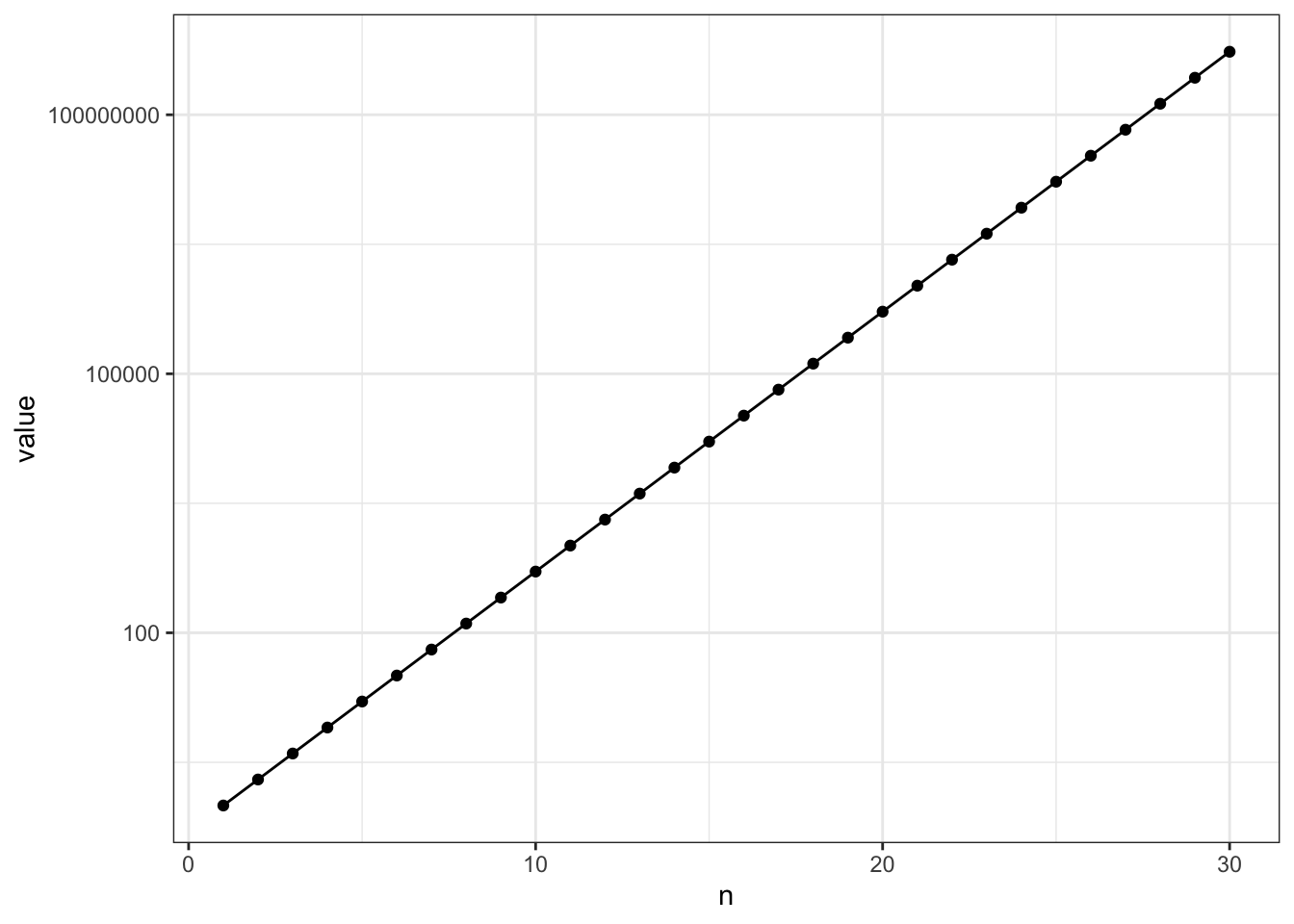With only one short change in the code, it seems like we have plotted a linear growth. But again, check the y-axis closely. The distance between to points on the axis is not as equal as the figure suggest. From step 1 to step 10, the difference is 1:512 (an increase of 511). However, from step 10 to step 20, 512:524,288 (an increase of 523,776!).

# Exponential growth based on percentages

Now in the case of the Corona virus spreading, we often quantify growth in percentage. So let’s consider another example. I now define a function that let’s us investigate different growth rates (based on percentages). So we again simulate a vector that represent exponential growth. This time, however, each value is the previous value plus a certain percentage of the previous value:

x_i = x_{i-1}*percent
``````perc_growth <- function(start = 1, steps, perc) {
x <- vector()
x <- start
for(i in 2:steps){
x[i] = x[i-1]+ perc*x[i-1]
}
return(x)
}

# Recreating the exponential growth from above
perc_growth(start = 1, steps = 10, perc = 1)``````
``##     1   2   4   8  16  32  64 128 256 512``

We see that if we start with 1 case and expect a 100% growth each day, we end up with 512 cases after 10 steps (e.g., 10 days). This is the exact exponential growth that we modelled above.

Of course, growth is not always 100%. It can be less, of course (but also more, like 200%!). In the next example, we see the effect of 5%, 10% and 20% growth on the growth curve.

``````(plot2 <- data.frame (n = 1:30,
"5" = perc_growth(start = 1, steps = 30, perc = 0.5),
"10" = perc_growth(start = 1, steps = 30, perc = 0.10),
"20" = perc_growth(start = 1, steps = 30, perc = 0.20)) %>%
gather(key, value, -n) %>%
ggplot(aes(x = n, y = value, color = key)) +
geom_point() +
geom_line() +
theme_bw())``````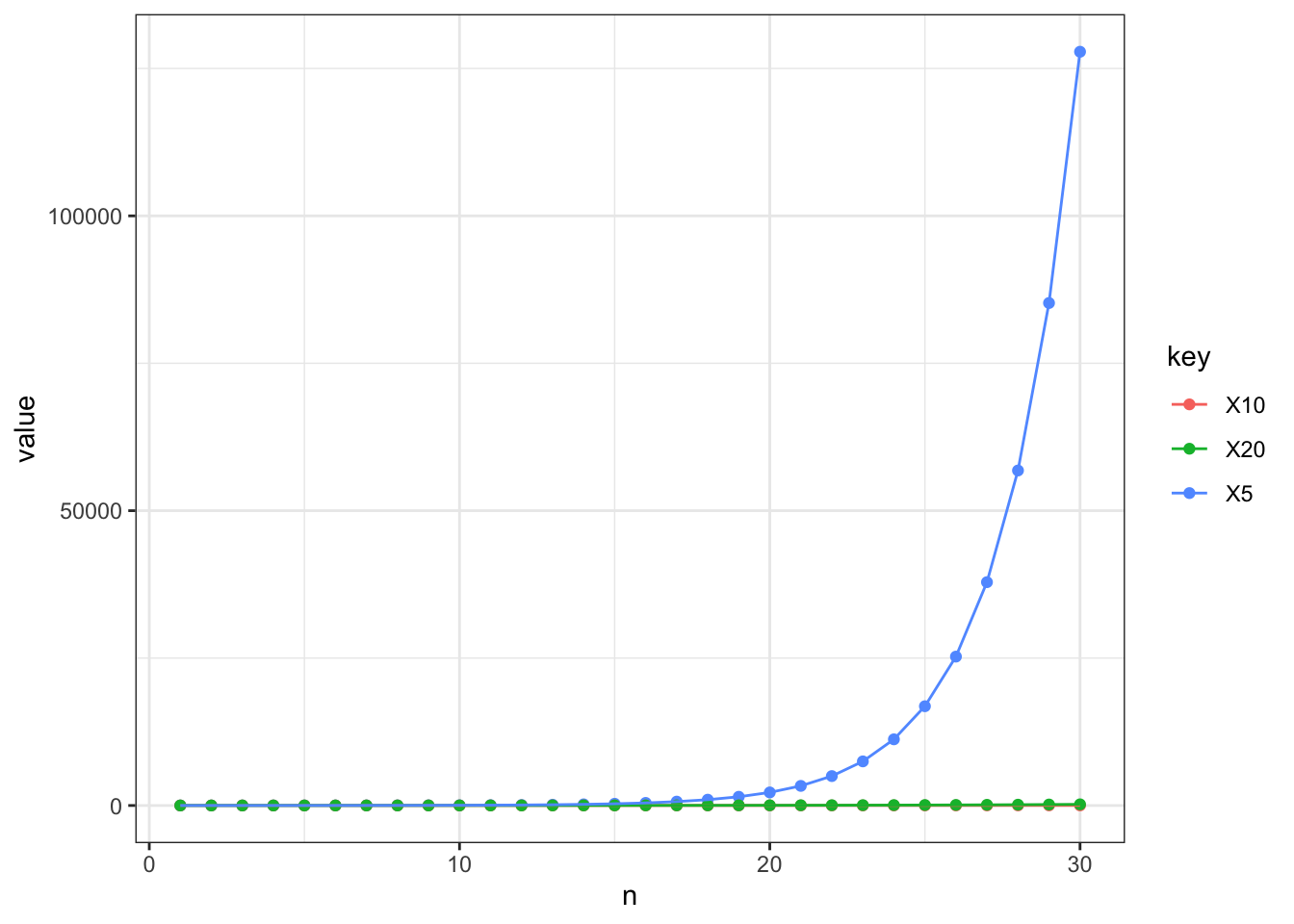Again we see that the growth of 50% is a lot steeper compared to 5% or 10%. Let’s again zoom in.

``````plot2 +
xlim(0, 10) +
ylim(0, 50)``````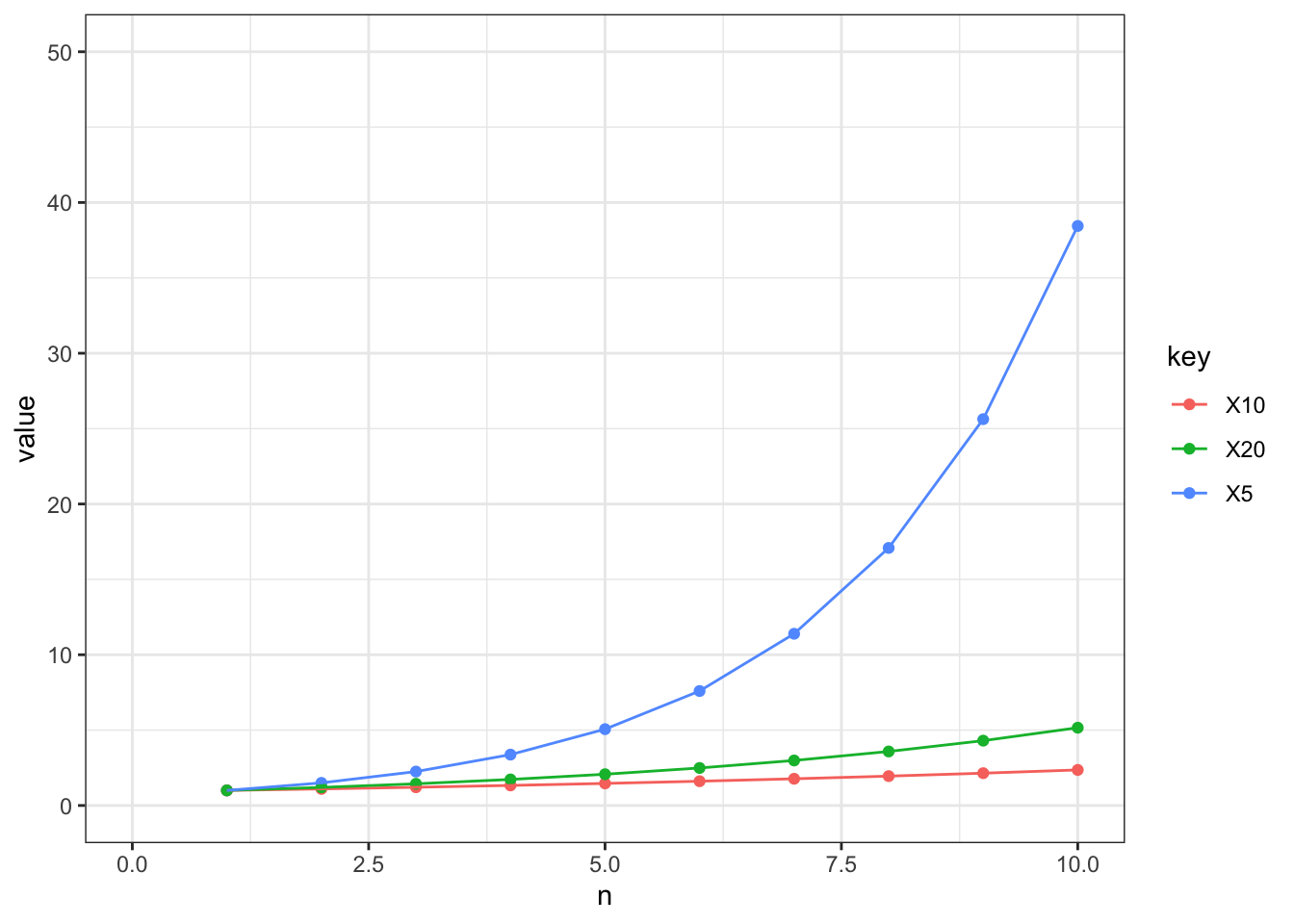As we can see, a 50% growth leads to ~40 cases after 10 steps (bear in mind, we started with only 1).

# Growth curves of the corona virus

Now let us visualize the growth curves of the corona virus infections per country. I therefore use the public data provided by the John Hopkins University (https://github.com/CSSEGISandData/COVID-19). For this purpose, I am only looking at the data from Germany.

``````d <- read.csv("data_new.csv") %>%
select(-Lat, -Long)

d <- d %>%
rename(country = Country.Region) %>%
filter(country == "Germany") %>%
gather(date, value, -country) %>%
separate(date, c("x", "date"), sep = 1) %>%
mutate(cases = as.numeric(value),
date = lubridate::mdy(date)) %>%
tbl_df

# plot
(plot3 <- d %>%
ggplot(aes(x = date, y = cases)) +
geom_point() +
geom_line() +
theme_bw() +
labs(x = "date", y = "cases"))``````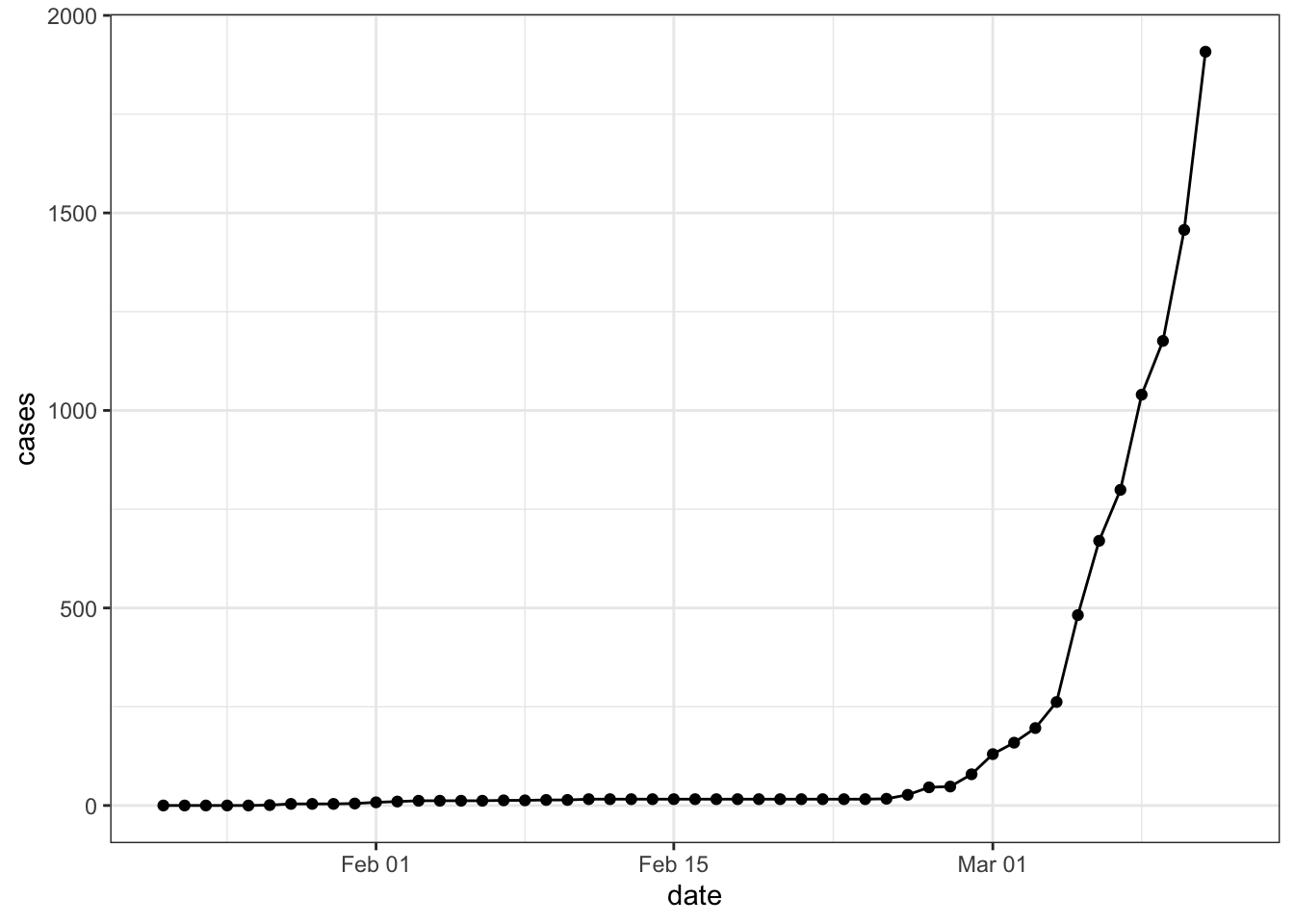If we compare this figure to the exponential growth curves that we simulated above, it should become clear that the actual growth curve of the corona pandemic in Germany is likewise exponential.

Again, if we log-transformed the y-axis and stretch the width of the figure (as shown in the tweet above), it again looks very different.

``````d %>%
ggplot(aes(x = date, y = cases + 1)) +
geom_point() +
geom_smooth(se = F, color = "black") +
theme_bw() +
scale_y_log10() +
labs(x = "date", y = "cases")``````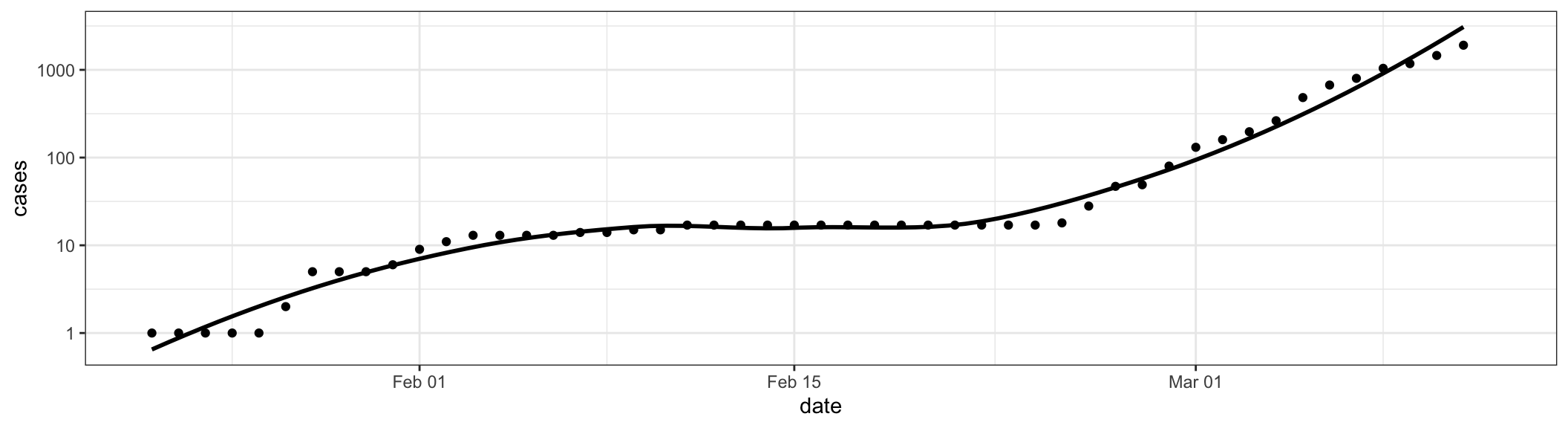But what is the actual growth rate (in percentage)? And what do we need to expect in the near future?

``````d %>%
mutate(cases_day = cases - lag(cases),
percent = cases_day/cases) %>%
summarize(growthrate = mean(percent, na.rm = T),
se = psych::describe(percent)\$se,
lower = growthrate - 1.96*se,
upper = growthrate + 1.96*se)``````
``````## # A tibble: 1 x 4
##   growthrate     se  lower upper
##        <dbl>  <dbl>  <dbl> <dbl>
## 1      0.154 0.0318 0.0918 0.216``````

The growth curve is on average ~15% (of course, we can debate if simply averaging across the growth rates per day is a good approach. Actually the figure in the tweet referenced above features a dotted line that represents a daily growth rate of 33% and most countries (including Germany) seem to approach that line). If we nonetheless use a growth rate of 15%, how would the increase in cases look like in a simulation for the next weeks and months?

Our starting value is 3,156 (the number of registered infections in Germany today).

``````data.frame(n = 1:30,
cases = perc_growth(start = 3156, steps = 30, perc = 0.154),
lower = perc_growth(start = 3156, steps = 30, perc = 0.0918),
upper = perc_growth(start = 3156, steps = 30, perc = 0.216)) %>%
gather(key, cases, -n) %>%
ggplot(aes(x = n, y = cases, color = key)) +
geom_line() +
theme_bw() +
ylim(0, 200000) +
scale_color_manual(values=c("black", "grey", "grey")) +
scale_x_continuous(breaks = seq(0,30, by = 5)) +
labs(x = "days", title = "Simulated growth in Germany")``````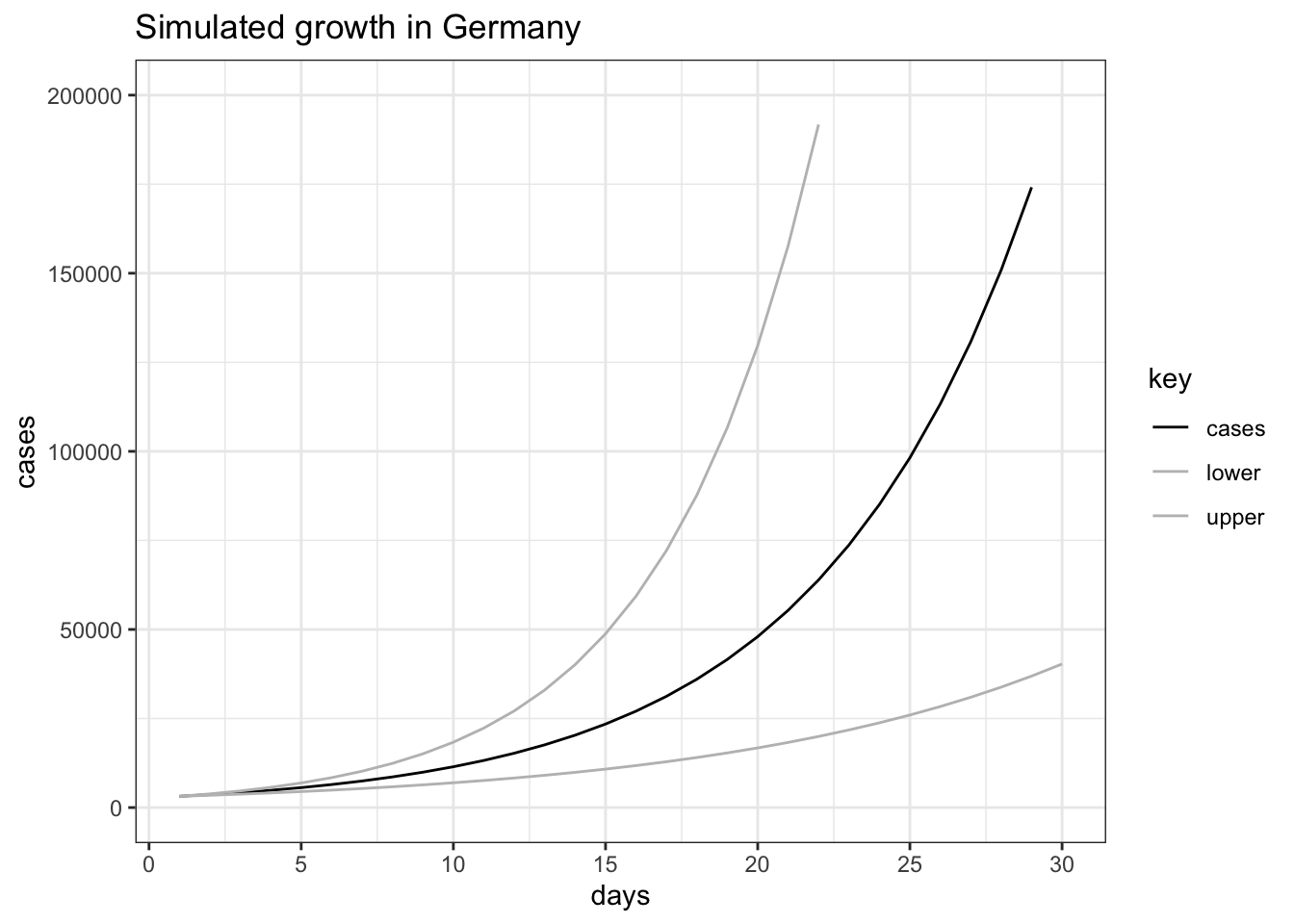The grey lines show the uncertainty in the simulation. The black curve shows the average growth rate based on the available data. It reveals that if the trend would continue, we would have more than 100,000 cases after only 25 days. Yet there are several things that have to be taken into account: First, the growth rate of 15% might be wrong (I admit that averaging across all days is a very crude way of estimating the growth rate) or may change over time. The large uncertainty (grey lines) shows already how largely the actual growth rate could vary based on my crude measure. For example, if the growth rate would be ~9%, we would have ~25,000 cases instead of ~100,000 after 25 days.

So how come that the news talk about a 33% growth curve now? Well, they only started to compute the growth rate after ~100 cases were identified. In the following figure, you see a simulated 33% growth curve plotted against the actual growth curve from the day when Germany had 100 identified cases.

``````(plot4 <- d %>%
filter(cases >= 100) %>%
mutate("cases of corona" = cases,
"33% growth curve" = perc_growth(start = 130, steps = 11, perc = .33)) %>%
select(date, "cases of corona", "33% growth curve") %>%
gather(key, value, -date) %>%
ggplot(aes(x = date, y = value, color = key)) +
geom_point() +
geom_line() +
theme_bw() +
scale_color_manual(values = c("grey", "black")) +
labs(x = "date", y = "cases", color = ""))``````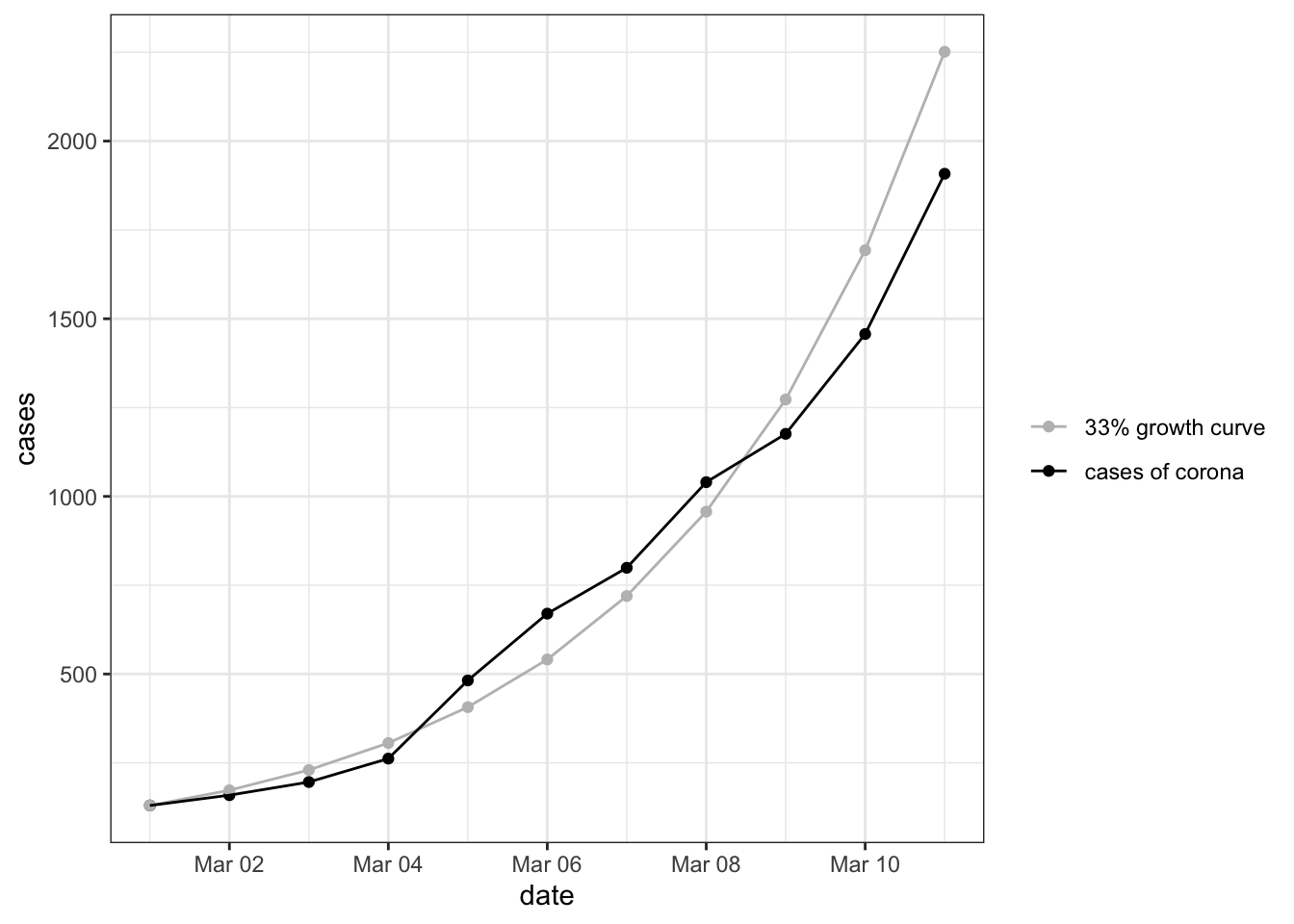As you can see, the curves align pretty well. If the daily growth rate would indeed approach 33% and stay this high for the next weeks, we would have ~2,961,940 cases after 25 days.

This is why acting now (both individually and on a societal level) is so important. We need to slow down this growth considerably. But we not only have to lower the actual infections, but also have to reduce the number of people who are infected at the same time. The latter is particularly important as the health system would otherwise not be able to treat all infected with the same care or even at all. The following video illustrates the effect of less infections at the same time on the health system very well:

# Conclusion

Understanding exponential growth is hard. The problem is that we constantly underestimate the actual growth and its implications. In case of infections, this is very worrisome. And I fear that figures that log-transformed the y-axis (such as the one presented above) might provide a false picture of the actual growth.

So what do we need to do? With no treatment and a viable vaccine yet available, the only effective way to keep the coronavirus pandemic at bay is to give the virus less chances of spreading. The following list of actions are recommended to serve as a set of guidelines:

1. Don’t panic, but be alert.
2. Wash your hands often and practice good cough and sneeze etiquette.
3. Try to touch your face as little as possible, including your mouth, nose, and eyes.
4. Practice social distancing, no hugs and kisses, no handshakes, no high fives. If you must, use safer alternatives.
5. Do not attend concerts, stage plays, sporting events, or any other mass entertainment events.
6. Refrain from visiting museums, exhibitions, movie theaters, night clubs, and other entertainment venues.
7. Stay away from social gatherings and events, like club meetings, religious services, and private parties.
8. Reduce your amount of travel to a minimum. Don’t travel long distances if not absolutely necessary.
9. Do not use public transportation if not absolutely necessary.
10. If you can work from home, work from home. Urge your employer to allow remote work if needed.
11. Replace as many social interactions as possible with remote alternatives like phone calls or video chat.
12. Do not leave your home if not absolutely necessary.

Be safe!